How to Learn in 24 Hours?The Rapid Learning Movie

 Need Help? M-F: 9am-5pm(PST): Toll-Free: (877) RAPID-10 US Direct: (714) 692-2900 Int'l: 001-714-692-2900 24/7 Online Technical Support: The Rapid Support Center Secure Online Order:Need Proof? Testimonials by Our Users

 Rapid Learning Courses: Chemistry in 24 Hours Biology in 24 Hours Physics in 24 Hours Mathematics in 24 Hours Psychology in 24 Hours SAT in 24 Hours ACT in 24 Hours AP in 24 Hours CLEP in 24 Hours MCAT in 24 Hours (Medical) USMLE in 24 Hours (Boards) DAT in 24 Hours (Dental) OAT in 24 Hours (Optometry) PCAT in 24 Hours (Pharmacy) Nursing Entrance Exams Certification in 24 Hours eBook - Survival Kits Audiobooks (MP3)

 Tell-A-Friend: Have friends taking science and math courses too? Tell them about our rapid learning system.Home »  Mathematics »  Introductory Statistics

Prerequisite Math Review

 Topic Review on "Title": Exponents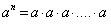Polynomial form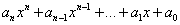Quadratic Equation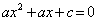Distance Formula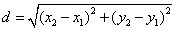Midpoint Formula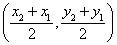Summation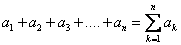Slope-Intercept Form   y = mx + b  Binomial Form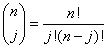Permutation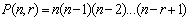Combination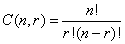Factorial n! = n(n-1)! Complex number a+bi  (a is real and b is imaginary part)

Rapid Study Kit for "Title":
 Flash Movie Flash Game Flash Card Core Concept Tutorial Problem Solving Drill Review Cheat Sheet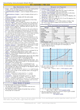"Title" Tutorial Summary : Prerequisite math is covered in this tutorial. This review is a bridging course for students who have neglected or forgotten their algebra. It will explain the principles of algebra and includes practice exercises with answers. This tutorial is designed to make the topic understandable and discussing natural numbers; integers; equations; factoring; algebraic fractions; radicals and exponents; quadratics; points, lines, and planes; and other topics.

 Tutorial Features: Specific Tutorial Features: Step by step mathematical examples showing all details: real numbers, geometry formulas, exponents, polynomials, factoring polynomials, rational expression, square roots, rational exponents, equations and other important formulas. Series Features: Concept map showing inter-connections of new concepts in this tutorial and those previously introduced. Definition slides introduce terms as they are needed. Visual representation of concepts Animated examples—worked out step by step A concise summary is given at the conclusion of the tutorial.

 "Title" Topic List: Real numbers Geometry formulas Exponents Polynomials Factoring polynomials Rational expression Square roots Rational exponents Linear equation Quadratic equations Solving inequalities Graphs and equations Distance formula Midpoint formula Slope-intercept form Lines Functions Complex numbers Sequences, summation, sets, permutation and combinations

See all 24 lessons in Introductory Statistics, including concept tutorials, problem drills and cheat sheets:
Teach Yourself Introductory Statistics Visually in 24 Hours